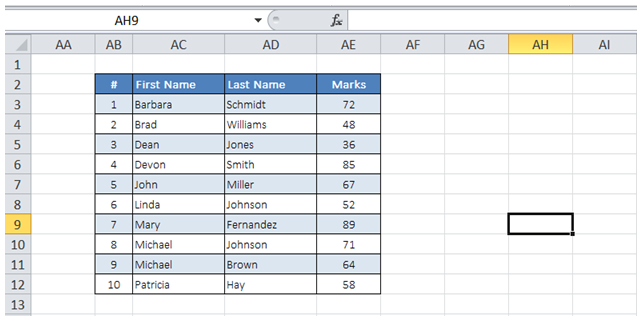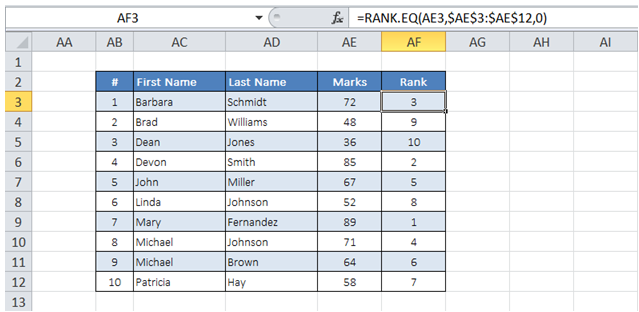## Rank of number in a list in Excel

For example, I need to determine the rank of students based on marks in the Table below.To do it in Excel, here is the answer:

a) Add a column for Rank as shown below.

Enter the formula =RANK.EQ(AE3,\$AE\$3:\$AE\$12,0) as shown below where AE3 corresponds to the cell containing the individual student's mark and \$AE\$3:\$AE\$12 corresponds to the range containing marks for all students.

There are 2 options to deal with the scenario when 2 students have the same mark (say both can be ranked 3)

- RANK.EQ (Both are assigned the same rank and next rank is skipped. In the example case, it would be 3 for both students and rank 4 is skipped in the list).

- RANK.AVG (Both are assigned the same average rank and both ranks are skipped. In the example case, it would be 3.5 for both students and ranks 3 and 4 are skipped in the list)

The third argument in the formula above "0" determines whether the ranking is in ascending order (1) or descending order (0).

b) Copy the formula for the rest of Rank Column.